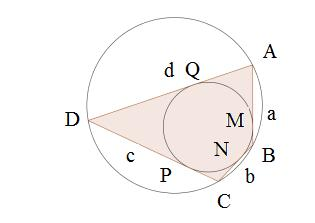#### You may also like### Some(?) of the Parts

A circle touches the lines OA, OB and AB where OA and OB are perpendicular. Show that the diameter of the circle is equal to the perimeter of the triangle### Polycircles

Show that for any triangle it is always possible to construct 3 touching circles with centres at the vertices. Is it possible to construct touching circles centred at the vertices of any polygon?### Circumspection

M is any point on the line AB. Squares of side length AM and MB are constructed and their circumcircles intersect at P (and M). Prove that the lines AD and BE produced pass through P.

##### Age 14 to 16Challenge Level
We received a great solution from Zhi of St. Marylebone CE School. She begins with a diagram:In the diagram presented, the vertices of the quadrilateral are labeled A, B, C and D, and tangent points M, N, P and Q respectively. The sides are labelled a, b, c and d.

From the properties of the circles we know that the tangents AM and AQ, BM and BN, CN and CP, DP and DQ are equal respectively. Therefore, if the quadrilateral is to be tangential, AQ+DQ+BN+CN would have to be equal to AM+DP+BM+CP, which then becomes:
$$AD+BC=AB+CD$$ Therefore, we know that a tangential quadrilateral has to satisfy AD+BC=AB+CD.

Using the properties of isosceles triangles and angle bisectors, we can deduce that the reverse is also true, meaning that all quadrilaterals satisfying $AD+BC=AB+CD$ are tangential. Is the reverse obvious? The question only asks for a necessary condition so in fact we do no need to show the reverse but this could be a nice extension.

The properties of cyclic quadrilaterals imply that for any cyclic quadrilateral ABCD, $\angle A+\angle C = \angle B +\angle D = 180^{\circ}$.The reverse is also true. Can you show this condition is necessary? See the problem Circles in Quadrilaterals.

Therefore, a bicentric quadrilateral ABCD has to meet two conditions
1. $AD+BD=AB+CD$ and
2. $\angle A + \angle C = \angle B +\angle D = 180 ^{\circ}$
With that , we can now deduce:
• Squares are always bicentric
• Rectangles and rhombi, provided that they aren't squares, cannot be bicentric as rectangles do not satisfy condition 1, and rhombi do not satisfy condtion 2.
• Irregular parallelograms do not meet either condition and cannot be bicentric
• Trapezia might or might not be bicentric, depending on their side lengths and angles.

Zhi then went on to prove the formula for the area of a bicentric quadrilateral. We shall omit the proof here since the question only asks you to verify that the formula works on the examples you found. If you are interested see the proof of brahmagupta's formula and try to adapt it to this problem.